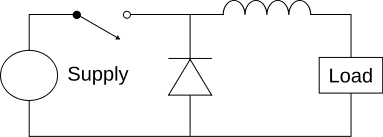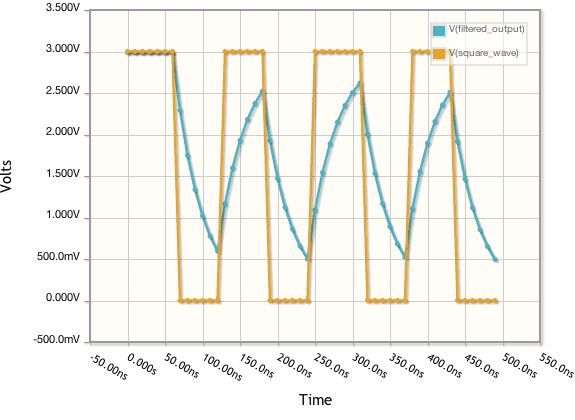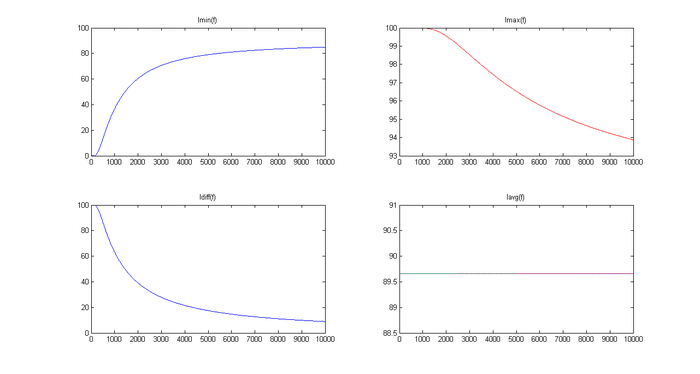# DC motor and switch frequency

• MechatronO
In summary, the author is looking for formulas to calculate different aspects of DC motors and buck converters. They have been unsuccessful in finding any information that meets their needs. They have come up with a formula for the max current through a switched RL circuit with a freewheeling diode(essentially a DC motor with the emf substracted from the input voltage), related to PWM period and duty. If someone is interested, they will post the derivation for this one and a similar formula for Imin.f

#### MechatronO

I've been looking around for formulas on how to calculate different aspects of DC motors and buck converters such as average current.

The reason is that I'm about to pick switch frequency for a motor, and don't know what characterics I can expect if I alter the switch frequency. Papers and publications regarding this are very much appreciated, as I haven't yet found any that answer my questions.

I succeded in coming up with a formula for the max current through a switched RL circuit with a freewheeling diode(essentially a DC motor with the emf substracted from the input voltage), related to PWM period and duty as

Imax = $\frac{E}{R}$ (1-e-RDT/L/(1-e-RT/L)

Where R is the circuits resistance, L is the inductance, D is the duty ranging from 0-1 and T is period time.

The circuit would look like this, with the load replaced by a resistor:The output current would look like the non-square wave in this diagram:I think its correct, at least the endpoints are as they should. If somebody is interested I'll can post the derivation for this one and a similar formula for Imin.

I was about to determine a formula for Iavg as well, however I decided to instead look for established info.

So, publications, formulas and personal experience regarding DC motor characteristics related to switch frequency are very much wanted. I'm especially interested in a formula that relates Iavg to switch frequency and duty cycle.

Seems like the biggest mystery in the world this switch frequency issue, have posted in two forums and nobody seem to know.

I tried to evalute

Iavg = 1/T∫i(t) dt

today, and came up with some rather clumpsy though exact expressions. As I couldn't simplify them enough to get an overview over the math, I used MATLAB to play around with it using the code below.

The result was surprising! No matter what switch frequency, the average current seemed to be constantly the same. Here is the resulting plot from the code below:Why doesn't physicsforum have their own image upload btw?

If my expressions below are correct, all that is needed is some playing around with the algebra and the result would be a simple expression for Iavg involving just D, R and L I think.

It would also be interesting to see the transisition times between steady state, related to switch frequency.

Code:
clear all
close all
clc % Clear Command window

L = 0.00001;    %Circuit inductance
R = 0.1;        %Circuit resistance
k=-R/L;         %To make the code more readable later

E=10;           %Supply voltage
D=0.9;          %Duty dycle

f= [10:10:10000] ;  %Switch frequencies
T=1./f;             %Associated periodtimes

imax = E/R*( 1-exp(k*D*T) )              ./  (1-exp(k*T))
imin = E/R*( exp(k*(T-D*T))-exp(k*T) )   ./  (1-exp(k*T));
deltai = imax-imin;

%Exact expressions for integral i(t) over one period i steady state, split in three parts
A1 = imin.*(exp(k*T)-1)/k ;
A2 = imax.*(exp(k*T)-exp(k*D*T))/k ;
A3 = E/R*(k*D*T-exp(k*D*T)+1)/k;

iavg = (A1+A2+A3)/T

%Plots
subplot(2,2,1);
plot(f,imin);
title('Imin(f)');

subplot(2,2,2);
plot(f,imax,'r');
title('Imax(f)');

subplot(2,2,3);
plot(f,deltai);
title('Idiff(f)');

subplot(2,2,4);
plot(f,iavg);
title('Iavg(f)');

The output plot is this:

Buck ckt - is Voltage Converter

IN the basic analysis Vout = Vin * Duty Cycle -- A buck ckt is a Voltage converter, and this is independent of Fsw.

Fsw is typically chosen for other factors: Losses and size of passives ( Magnetics and Caps), Ripple ( V and I) to the load, EMI, Audible noise, interference with other devices, etc...

The price for the higher Fsw is the switch and diode losses are increased. -- See the attached basic simulation - sorry I don't work with 1.5 V ! --- The good info here are the optional Characteristic plots at the end of the report. This is for sizing the power electronics - so the "limits" shown are based on maximum device temperatures - NOT the current changing based on the Fsw. For example at 20kHz the device can only handle 2/3 of the current that it can at 10kHz without overheating. - For a given load the current would be same as Fsw changes, but at 10kHz the dsvice can handle more load.

Lastly - MOSFETs do not have as high of switching losses as IGBTs - so the effect is much less pronounced, and Fsw of 300kHz - for small power are not uncommon.

#### Attachments

• Buck Ckt for Physics Forums.pdf
344 KB · Views: 354
There are switching regulator simulation programs that let you determine all the efficiency issues and waveforms for inductive switching circuits of just about any topology. LTSpice is one. http://www.linear.com/designtools/software/